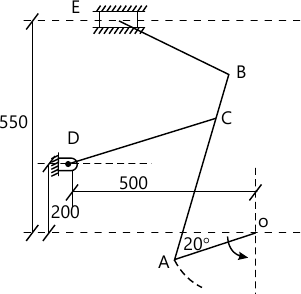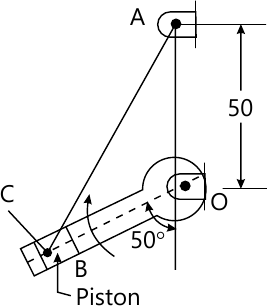MORE IN Theory of Machines - 1
MU Mechanical Engineering (Semester 4)
Theory of Machines - 1
December 2013
Total marks: --
Total time: --
INSTRUCTIONS
(1) Assume appropriate data and state your reasons
(2) Marks are given to the right of every question
(3) Draw neat diagrams wherever necessary

Explain any four of the following questions-
1 (a) State and prove Kennedy's Theorem.
5 M
1 (b) Any one of the exact straight line generating mechanism.
5 M
1 (c) Chordal action in chains
5 M
1 (d) D Alembert's principle to bodies under rotational motion about a fixed axis.
5 M
1 (e) Law of gearing with neat sketch.
5 M

2 (a) Fig 1 shows the mechanism of a radial valve gear. Crank OA rotates uniformly at 150 rpm and is pinned at A to rod AB, Point C is guided in the circular path with D as centre and DC as radius. Dimensions of various links are- OA=150 mm; AB=550 mm; AC=450; DC=500 mm; BE=350 mm. Determine the velocity of the slider at E by-
i) Relative velocity method.
ii) Instantaneous centre method.12 M
2 (b) Draw a neat sketch of a 'Tehebicheff' mechanism and prove that the lengths of the link must be in a ratio of 1:2:2.5 for a point on the coupler to trace an approximate straight line.
8 M

3 (a) What is the condition for correct steering? Discuss the relative advantages of Davis over the Ackermann steering mechanism.
8 M
3 (b) The kinematic diagram of one of the cylinders of a rotary engine is the fig 2. The crank OA which is vertical and fixed is 50 mm long. The length of the connecting rod AB is 125 mm. The line of the stroke OB is inclined at 50° to the vertical. The cylinders are rotating at a uniform speed of 300 rpm in a clockwise direction, about the fixed centre O. Determine:-
i) Acceleration of the piston inside the cylinder
ii) Angular acceleration of the connecting rod12 M

4 (a) The following data relate to a connecting rod of a reciprocating engine. Mass=55kg; Distance between bearing centre=850 mm; Diameter of small end bearing=75; Diameter of big end bearing=100 mm. Time oscillation when the connecting rod is suspended from small end=1.83 sec, Time of oscillation when the connecting rod is suspended from big end=1.68 sec.
Determine-
i) The radius of gyration of the rod about an axis passing through the centre of gravity and perpendicular to the plane of oscillation.
ii) The moment of inertia of the rod about the same axis.
iii) The dynamically equivalent system for the connecting rod, constituted of two masses, one of which is situated at the small end centre.
14 M
4 (b) Explain work energy principle and conservation of energy.
6 M

5 (a) A punching press is required to punch 30mm diameter hole in a plate of 20mm thickness at the rate of 20 holes per minute. It required 6 N-M of energy/mm2 of shared area. If punching takes place in I/10 of a second and the rpm of the flywheel varies from 160 to 140, determine the weigh of flywheel having radius of gyration k=1m.
10 M
5 (b) A v-belt having face width equal to 22 mm and nominal thickness equal to 14mm is used to transmit power with 'V' groove angle 40°. If the mass of the belt is 0.4 kg/m and maximum allowable stress is 1.5 N/mm2, determine the maximum power that can be transmitted. Angle of contact is 155° and co-efficient of friction is 0.2.
10 M

6 (a) The centre to centre distance between the two sprockets of a chain drive is 600mm. The chain drive is used to reduce the speed form 180 rpm to 90 rpm. The driving sprocket has 18 teeth and a pitch circle of 480 mm
Determine:-
i) Number of teeth on the driven sprocket
ii) Pitch and the length of chain.
10 M
6 (b) Derive an expression for approximate length of an open belt drive.
10 M

7 (a) Two mating gears have 20 and 40 teeth of module 10mm and 20° pressure angle. The addendum of each wheel is to be made of such a length that the line of contact on each side of the pitch point has half the maximum possible length. Determine the addendum height for each gear wheel, length of path of contact, arc of contact and contact ratio.
10 M
7 (b) Two 20° involute spur gear mesh externally and give a velocity ratio of 3. Module is 3 mm and the addendum is equal to 1.1 module. If the pinion rotates at 120 rpm, determine.
i) The minimum number of teeth on each wheel to avoid interference.
ii) The number of pair of teeth in contact.
10 M

More question papers from Theory of Machines - 1